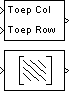# Toeplitz

Generate matrix with Toeplitz symmetry

## Library

Math Functions / Matrices and Linear Algebra / Matrix Operations

`dspmtrx3`

•## Description

The Toeplitz block generates a Toeplitz matrix from inputs defining the first column and first row. The top input (`Col`) is a vector containing the values to be placed in the first column of the matrix, and the bottom input (`Row`) is a vector containing the values to be placed in the first row of the matrix.

```y = toeplitz(Col,Row) % Equivalent MATLAB code ```

The other elements of the matrix obey the relationship

```y(i,j) = y(i-1,j-1) ```

and the output has dimension `[length(Col) length(Row)]`. The y(1,1) element is inherited from the `Col` input. For example, the following inputs

```Col = [1 2 3 4 5] Row = [7 7 3 3 2 1 3] ```

produce the Toeplitz matrix

`$\left[\begin{array}{ccccccc}1& 7& 3& 3& 2& 1& 3\\ 2& 1& 7& 3& 3& 2& 1\\ 3& 2& 1& 7& 3& 3& 2\\ 4& 3& 2& 1& 7& 3& 3\\ 5& 4& 3& 2& 1& 7& 3\end{array}\right]$`

When you select the Symmetric check box, the block generates a symmetric (Hermitian) Toeplitz matrix from a single input, `u`, defining both the first row and first column of the matrix.

```y = toeplitz(u) % Equivalent MATLAB code ```

The output has dimension `[length(u) length(u)]`. For example, the Toeplitz matrix generated from the input vector `[1 2 3 4]` is

`$\left[\begin{array}{cccc}1& 2& 3& 4\\ 2& 1& 2& 3\\ 3& 2& 1& 2\\ 4& 3& 2& 1\end{array}\right]$`

The Toeplitz block supports real and complex floating-point and fixed-point inputs.

## Parameters

Symmetric

When selected, enables the single-input configuration for symmetric Toeplitz matrix output.

Saturate on integer overflow

When you generate a symmetric Toeplitz matrix with this block, if the input vector is complex, the output is a symmetric Hermitian matrix whose elements satisfy the relationship

`$y\left(i,j\right)=\mathrm{conj}\left(y\left(j,i\right)\right)$`

For fixed-point signals the conjugate operation could result in an overflow. When you select this parameter, overflows saturate. This parameter is only visible with the Symmetric parameter is selected. This parameter is ignored for floating-point signals.

## Supported Data Types

PortSupported Data Types

Input

• Double-precision floating point

• Single-precision floating point

• Fixed point (signed and unsigned)

• Boolean

• 8-, 16-, and 32-bit signed integers

• 8-, 16-, and 32-bit unsigned integers (real signals only)

Toep Col

• Double-precision floating point

• Single-precision floating point

• Fixed point (signed and unsigned)

• Boolean

• 8-, 16-, and 32-bit signed integers

• 8-, 16-, and 32-bit unsigned integers

Toep Row

• Double-precision floating point

• Single-precision floating point

• Fixed point (signed and unsigned)

• Boolean

• 8-, 16-, and 32-bit signed integers

• 8-, 16-, and 32-bit unsigned integers

Output

• Double-precision floating point

• Single-precision floating point

• Fixed point (signed and unsigned)

• Boolean

• 8-, 16-, and 32-bit signed integers

• 8-, 16-, and 32-bit unsigned integers

 `toeplitz` MATLAB

## Extended Capabilities

### Fixed-Point ConversionDesign and simulate fixed-point systems using Fixed-Point Designer™.

Introduced before R2006a

## SupportGet trial now